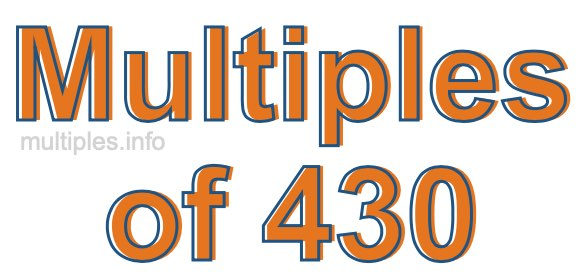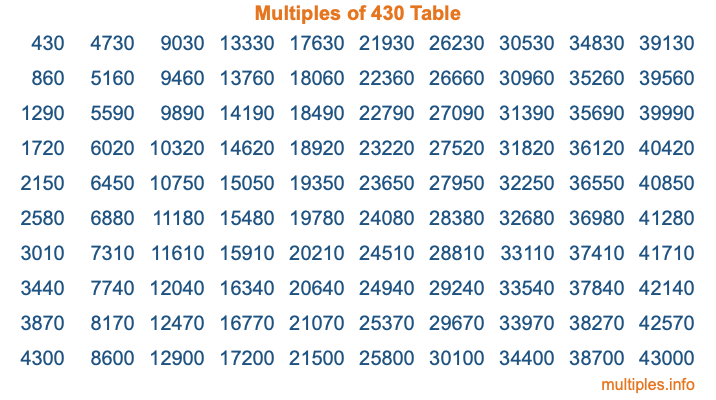Multiples of 430Welcome to the Multiples of 430 page. Here we will first teach you everything you will ever need to know about the multiples of 430, and then give you a study guide summary of everything we taught you to make sure you remember it all. Use this page to look up facts and learn information about the multiples of 430. This page will make you a multiples of four hundred thirty expert!

Definition of Multiples of 430
Multiples of 430 are all the numbers that when divided by 430 equal an integer. Each of the multiples of 430 are called a multiple. A multiple of 430 is created by multiplying 430 by an integer.

Therefore, to create a list of multiples of 430, you start with 1 multiplied by 430, then 2 multiplied by 430, then 3 multiplied by 430, and so on for as long as you want. Thus, the list of the first five multiples of 430 is 430, 860, 1290, 1720, and 2150. To see a larger list of multiples of 430, see the printable image of Multiples of 430 further down on this page. We also have a category where you can choose any nth multiple of 430.

Multiples of 430 Checker
The Multiples of 430 Checker below checks to see if any number of your choice is a multiple of 430. In other words, it checks to see if there is any number (integer) that when multiplied by 430 will equal your number. To do that, we divide your number by 430. If the the quotient is an integer, then your number is a multiple of 430.

Is  a multiple of 430?

Least Common Multiple of 430 and ...
A Least Common Multiple (LCM) is the lowest multiple that two or more numbers have in common. This is also called the smallest common multiple or lowest common multiple and is useful to know when you are adding our subtracting fractions. Enter one or more numbers below (430 is already entered) to find the LCM.

Check out our LCM Calculator if you need more details about the Least Common Multiple or if you need the LCM for different numbers for adding and subtraction fractions.

nth Multiple of 430
As we stated above, 430 is the first multiple of 430, 860 is the second multiple of 430, 1290 is the third multiple of 430, and so on. Enter a number below to find the nth multiple of 430.

th multiple of 430

Multiples of 430 vs Factors of 430
430 is a multiple of 430 and a factor of 430, but that is where the similarities end. All postive multiples of 430 are 430 or greater than 430. All positive factors of 430 are 430 or less than 430.

Below is the beginning list of multiples of 430 and the factors of 430 so you can compare:

Multiples of 430: 430, 860, 1290, 1720, 2150, etc.

Factors of 430: 1, 2, 5, 10, 43, 86, 215, 430

As you can see, the multiples of 430 are all the numbers that you can divide by 430 to get a whole number. The factors of 430, on the other hand, are all the whole numbers that you can multiply by another whole number to get 430.

It's also interesting to note that if a number (x) is a factor of 430, then 430 will also be a multiple of that number (x).

Multiples of 430 vs Divisors of 430
The divisors of 430 are all the integers that 430 can be divided by evenly. Below is a list of the divisors of 430.

Divisors of 430: 1, 2, 5, 10, 43, 86, 215, 430

The interesting thing to note here is that if you take any multiple of 430 and divide it by a divisor of 430, you will see that the quotient is an integer.

Multiples of 430 Table
Below is an image of the first 100 multiples of 430 in a table. The table is in chronological order, column by column. The first column has the first ten multiples of 430, the second column has the next ten multiples of 430, and so on.The Multiples of 430 Table is also referred to as the 430 Times Table or Times Table of 430. You are welcome to print out our table for your studies.

Negative Multiples of 430
Although not often discussed or needed in math, it is worth mentioning that you can make a list of negative multiples of 430 by multiplying 430 by -1, then by -2, then by -3, and so on, to get the following list of negative multiples of 430:

-430, -860, -1290, -1720, -2150, etc.

Multiples of 430 Summary
Below is a summary of important Multiples of 430 facts that we have discussed on this page. To retain the knowledge on this page, we recommend that you read through the summary and explain to yourself or a study partner why they hold true.

There are an infinite number of multiples of 430.

A multiple of 430 divided by 430 will equal a whole number.

430 divided by a factor of 430 equals a divisor of 430.

The nth multiple of 430 is n times 430.

The largest factor of 430 is equal to the first positive multiple of 430.

430 is a multiple of every factor of 430.

430 is a multiple of 430.

A multiple of 430 divided by a divisor of 430 equals an integer.

430 divided by a divisor of 430 equals a factor of 430.

Any integer times 430 will equal a multiple of 430.

Multiples of a Number
Here you can get the multiples of another number, all with the same attention to detail as we did for multiples of 430 on this page.

Multiples of
Multiples of 431
Did you find our page about multiples of four hundred thirty educational? Do you want more knowledge? Check out the multiples of the next number on our list!